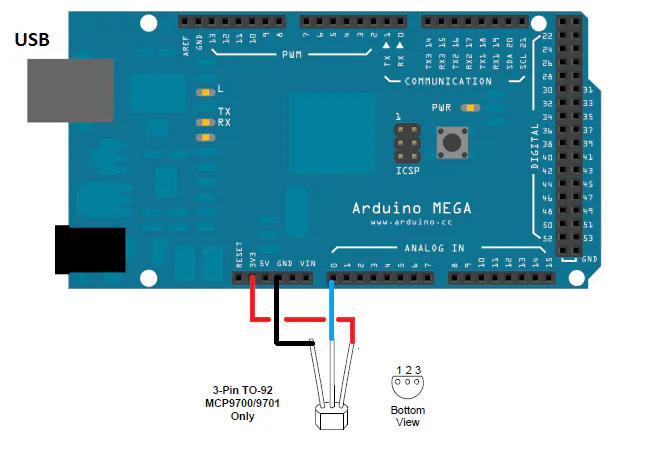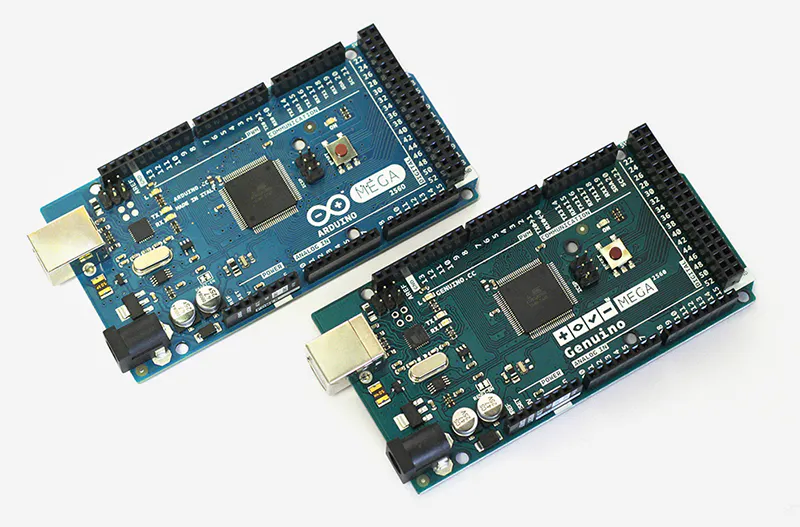# Active Sensor Thermometer

How to build a simple and accurate thermometer using an active temperature sensor (MCP9071/A).

IntermediateProtip1 hour4,638## Things used in this project

### Hardware componentsArduino Mega 2560 & Genuino Mega 2560
×1
 Microchip Technology MCP9071/A
×1

## Schematics

### SCHEMATIC

The hardware implementation is executed by using the Arduino MEGA 2560 card: just connect the active sensor as shown in the figure. Pin A0 is set as an analog input for converting the output voltage of the sensor to the AD.## Code

### Arduino code for thermometer active sensor based

Arduino
taking the application scheme as referencehow to connect the sensor to the Arduino board and loading this code in the arduino, it is possibile read the room temperature in the serial output interface. Every 1s the measure is done and printed
```/*

The value of Vout is obtained by averaging 1024 values reading the voltage on pin A0. whicch is connected to the sensor output. The value of temeprature ta, read by the sensor is shown on the serial monitor.

*/

// set pin numbers:

const int in = A0;            // input for reading the sensor output

// set constants:
const float vout0 = 400;   //  sensor output voltage in mV at 0°C
const float tc = 19.53;   // mV for °C temperature constant for the  MCP9701/A

// variables:

int i, f;
float vout, vout_avg, ta;

// MAIN PROGRAM

void setup() {
Serial.begin(9600);
pinMode(in, INPUT);            // set pin in as input
pinMode(13, OUTPUT);            // set pin 13 as output
digitalWrite(13, LOW);
analogReference(DEFAULT);

}

void loop() {
vout_avg = 0;
for (i = 0; i < 1024; i++) {
vout = analogRead(A0) * (4976.30 / 1023);
//Serial.println(vout);
vout_avg = vout_avg + vout;
}
vout = vout_avg / 1024;
//Serial.println(vout);
ta = (vout - vout0) / tc;
Serial.print("temperature ( celcius degree)   = " );
Serial.println(ta);
delay (1000);
}
```

## Credits

### microst

9 projects • 7 followers
Electronic is my life passion...